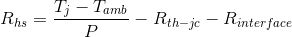# Heat Sink Thermal Resistance Calculator

Use this online calculator to determine the heat sink thermal resistance required to maintain a specified component junction temperature.

The resistance to heat flow from the junction of the heat generating component, through the casing, thermal interface material, heat sink and finally to environment is represented by the thermal resistance circuit shown in the diagram below. The following equation is used to calculate Rhs, the thermal resistance of the heat sink:Where:

Tj
is the junction temperature
Tamb
is the ambient temperature
P
is the heat dissipated from the heat source
Rth-jc
is the junction to case thermal resistance
Rinterface
is the thermal interface material resistance calculated using the following equation:tinterface
is the thermal interface material thickness:
kinterface
is the thermal conductivity of interface material
Ls
is the length of heat source:
Ws
is the width of heat source
°C
°C
Watts
Thermal interface material
mm
Watts/(m⋅K)
mm
mm
Junction to case thermal resistance Rth-jc:
C/W## Do you need more functionality?

The HeatSinkCalculator offers online heat sink analysis software that allows you to analyze plate fins heat sinks cooled via radiation, and natural or forced convection. Multiple heat sources attached to the heat sink can be evaluated. In addition the calculator automatically determines the optimal dimensions of your heat sink to achieve the lowest heat source temperature. Click below to learn more.